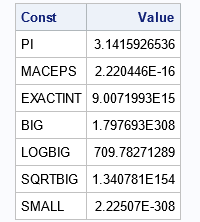Statistical programmers need to access numerical constants that help us to write robust and accurate programs. Specifically, it is necessary to know when it is safe to perform numerical operations such as raising a number to a power without exceeding the largest number that is representable in finite-precision arithmetic. This article discusses five constants that every statistical programmer must know: PI, MACEPS, EXACTINT, BIG, and SMALL. In the SAS language, you can find the values of these constants by using the CONSTANT function in Base SAS.

The following table shows the values of the constants that are discussed in this article:

```data Constants; length Const \$10; format Value Best12.; input Const @@; Value = constant(Const); datalines; PI MACEPS EXACTINT BIG LOGBIG SQRTBIG SMALL ;   proc print noobs; run;```### Pi and other mathematical constants

The CONSTANT function provides values for various mathematical constants. Of these, the most important constant is π ≈ 3.14159..., but you can also obtain other mathematical constants such as the natural base (e) and the golden ratio (φ). To get an approximation for π, use pi = constant('pi'). The number π is essential for working with angles and trigonometric functions. For an example, see the article, "Polygons, pi, and linear approximations," which uses π to create regular polygons.

### Machine epsilon

Arguably the most important constant in computer science is machine epsilon. This number enables you to perform floating-point comparisons such as deciding whether two numbers are equal or determining the rank of a numerical matrix. To get machine epsilon, use eps = constant('maceps').

### The largest representable integer

It is important to know the largest number that can be represented accurately in finite precision on your machine. This number is available as bigInt = constant('exactint'). This number enables you to find the largest factorial number that you can compute (18!) and the largest row of Pascal's triangle (56) that you can faithfully represent in double precision (56).

### The largest representable double

Except for π, the constants I use the most are related to BIG ≈ 1.8E308. I primarily use LOGBIG and SQRTBIG, which you can compute as
logbig = constant('logbig');
sqrtbig = constant('sqrtbig');
These constants are useful for preventing overflow when you perform arithmetic operations on large numbers:

• The quantity exp(x) can only be computed when x is less than LOGBIG ≈ 709.78.
• The LOGBIG option supports the BASE suboption (BASE > 1), which you can use to ensure that raising a number to a power does not overflow. For example, constant('logbig', 2) returns 1024 because 2**1024 is the largest power of 2 that does not exceed BIG.
• The SQRTBIG option tells you whether you can square a number without overflowing.

### The smallest representable double

What is the smallest positive floating-point number on your computer? It is given by small = constant('small'). There are also LOGSMALL and SQRTSMALL versions, which you can use to prevent overflow. I don't use these constants as frequently as their 'BIG' counterparts. In my experience, underflow is usually not a problem in SAS.

### Summary

This article discusses five constants that every statistical programmer must know: PI, MACEPS, EXACTINT, BIG, and SMALL. Whether you need mathematical constants (such as π) will depend on the programs that you write. The MACEPS constant is used to compare floating-point numbers. The other constants are used by computer scientists and numerical analysts to ensure that programs can correctly compute with very large (or very small) numbers without encountering floating-point overflow (or underflow).

The post Five constants every statistical programmer should know appeared first on The DO Loop.